# Wave Packet Solution

Since the traveling wave solution to the wave equation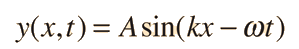is valid for any values of the wave parameters, and since any superposition of solutions is also a solution, then one can construct a wave packet solution as a sum of traveling waves: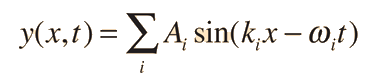It is common practice to use to represent the quantity 2π/λ by k, which is called the wave vector. For a continuous range of wave vectors k, the sum is replaced by an integral: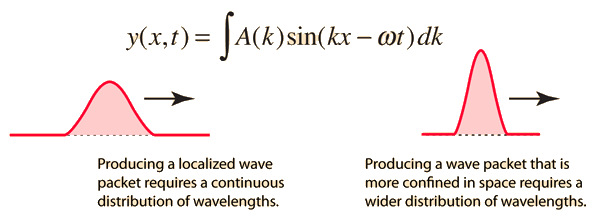Wave packet details
Index

Wave concepts

Reference
Tipler
Elementary Modern Physics, Appendix B

 HyperPhysics***** Sound R Nave
Go Back

# Wave Packet Details

A wave packet solution to the wave equation, like a pulse on a string, must contain a range of frequencies. The shorter the pulse in time, the greater the range of frequency components required for the fast transient behavior. This requirement can be stated as a kind of uncertainty principle for classical waves: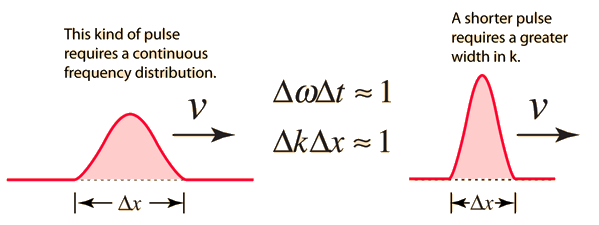The actual numbers involved depend upon the definition of the pulse width, but creating short pulses inherently requires a large frequency bandwidth. This is similar in nature to the uncertainty principle in quantum mechanics.

 Compare with discrete frequencies
Index

Wave concepts

 HyperPhysics***** Sound R Nave
Go Back

# Wave Packets from Discrete Waves

If discrete traveling wave solutions to the wave equation are combined, they can be used to create a wave packet which begins to localize the wave. This property of classical waves in mirrored in the quantum mechanical uncertainty principle.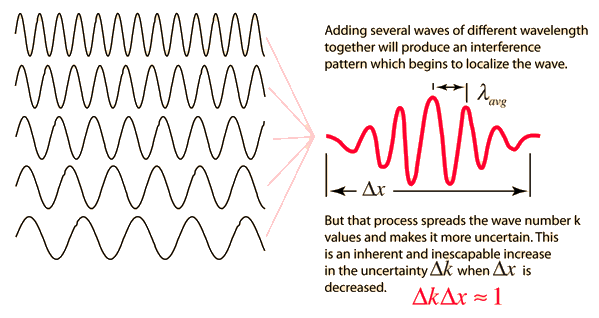Index

Wave concepts

 HyperPhysics***** Sound R Nave
Go Back